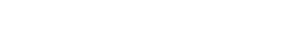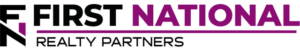# Accelerated Depreciation Definition for Investors

### Key Takeaways

• Depreciation is an accounting concept that allows asset owners to “expense” a portion of the asset’s value to account for its deterioration over time.
• Depreciation is a non-cash expense that appears on the income statement and it serves to reduce the amount of taxable income that a business produces each year.
• As a result, business owners are strongly incentivized to take as much depreciation as legally possible.
• There are a number of ways to “accelerate” the amount of depreciation taken for fixed assets.  Common methods include double declining balance, sum of years, and cost segregation.
• In a real estate context, accelerated depreciation reduces the amount of taxable income that is passed on to investors, reducing their overall tax liability.  This is a significant benefit of commercial real estate investment.

One of the most commonly cited benefits of a commercial real estate investment is the potential tax savings that comes with it. To this point, there are a number of different ways that an investor can reduce their taxes with a real estate investment, but one of the most important is known as “depreciation.”

In this article, we are going to describe what depreciation is, how it works, why it matters, and how it can be “accelerated” to maximize tax savings. By the end, the goal is for readers to have a more thorough understanding of what accelerated depreciation is and how it can be incorporated into their pre-purchase due diligence process.

At First National Realty Partners, we specialize in the purchase and management of grocery store anchored retail centers. As part of our management effort, we seek to maximize depreciation for all of our properties in an effort to maximize tax savings on behalf of our investors. If you are an Accredited Investor and would like to learn more about our current investment opportunities, click here.

## What is Depreciation?

In order to understand what Accelerated Depreciation is, it is first helpful to understand depreciation as a concept.

Depreciation is a non-cash line item on the income statement that allows owners to “expense” a portion of an asset’s value each year to account for its physical deterioration over time. In a real estate context, the depreciation expense line item is meant to account for the decline in the physical condition of a property. For example, a building’s roof wears out over time and there is a cost associated with this, which is what the depreciation line item attempts to capture.

There are a number of ways that depreciation can be calculated, but the most simplistic is known as “straight-line depreciation.” With this method of depreciation, an asset’s value is divided by the number of years in its “useful life.” In a very simple example, suppose that a property has a value of \$1MM and an estimated useful life of 30 years. In this case, the owner would be able to “expense” \$33,333 in depreciation each year.

## Why Does It Matter?

Depreciation matters because it reduces the amount of taxable income produced by a property. To illustrate this point, consider a very simple example.

In one scenario, suppose that a property produces \$100,000 in annual rental income and \$25,000 in operating expenses – without depreciation. As such, the resulting Net Operating Income (NOI) is \$75,000. If debt service is \$25,000, cash flow before taxes is \$50,000.

Now consider a scenario with depreciation. The property produces the same \$100,000, but operating expenses are \$50,000- which includes \$25,000 in depreciation. The resulting Net Operating Income is \$50,000. After \$25,000 in debt service, the resulting cash flow before tax is \$25,000. But, here is the key point in this scenario, depreciation is a non-cash expense, which means that it reduces taxable income, but not the amount of cash available for distribution.

Because of the way that accounting rules treat depreciation, owners are incentivized to maximize it.

## So, What Is Accelerated Depreciation?

Accelerated Depreciation occurs when property owners utilize certain rules to increase the amount of depreciation taken each year. It is considered to be “accelerated” because it is more than would normally be allowable under standard, straight line depreciation rules. Or, it may be “bonus depreciation” that is allowed on top of normal straight line depreciation.

There are a number of ways that property owners can accelerate depreciation.

### Bonus Depreciation & Section 179 Deductions

Under IRS rules for bonus depreciation, property owners are allowed to deduct 100% of the cost of capital improvements in the year in which the expense is incurred. If the depreciation is enough, it may create a net loss. For example, we are value-add investors, which means that we like to buy properties and invest in things like landscaping, building facades, and parking lots. The cost of these improvements can be deducted in the first year in which they are incurred, which can create a significant benefit for our investors for tax purposes.

Section 179 deductions also allow investors to deduct the cost of certain types of improvements and equipment in the year in which they are incurred. However, the key difference is that the deduction is limited to the amount of taxable income. In other words, it cannot create a loss.

Both of these types of depreciation are useful for the purchase of fixed assets and the reduction of income taxes.

### Double Declining Balance Method of Depreciation

The “double declining balance” method of accelerating depreciation allows property owners to depreciate the balance on certain assets twice as fast. The math can be complex, but an example is provided below.

Suppose that a property owner replaced a roof at a cost of \$1MM and it had an estimated useful life of 30 years. Under the straight-line depreciation method, the depreciation rate would be \$33,333 per year.

Under the double declining balance method, the depreciation rate is calculated as 2 times the straight line depreciation percent times the book value of the asset at the beginning of the period. So, for example, the straight line depreciation percentage is (1/30) 3.33% annually. Under the double declining balance, the depreciation rate would be (2 X 3.33%) or 6.66% multiplied by the book value of \$1,000,000 in the first year. So, the first year of depreciation would be (6.66% x \$1,000,000) or \$66,666 in the first year. In the second year, it would be 6.66% multiplied by (\$1,000,000 – \$66,666) \$933,333 or \$62,160. The balance would continue to “decline” until the value of the asset reached its estimated salvage value.

The bottom line is this, the double declining balance accelerated depreciation method is a way to take more depreciation and reduce the property’s tax liability in the process.

### Sum of the Year’s Digits (SYD)

Sum of the Year’s Digits is another accelerated method of depreciation that sums the number of digits in the useful life of an asset to calculate the annual depreciation amount.

For example, suppose that an asset has an estimated useful life of five years. The sum of the years’ digits method is calculated as 5 + 4 + 3 + 2 + 1 = 15. Then, to calculate the amount of depreciation for each year, the number of years remaining in the useful life is divided by the sum of the years digits. So, in year one, depreciation would be 5/15 or 33%. In year 2, it would be 4/15 or 26.6% and so on until the value of the asset is fully depreciated.

This depreciation method is especially useful for assets whose values reduce rapidly in the early years of ownership – like an automobile.

### Cost Segregation

As it relates specifically to commercial real estate, the cost segregation accounting method of depreciation is one of the more common ways to accelerate depreciation.

To help understand how cost segregation works, it is helpful to think of a commercial property as a series of depreciable assets like mechanical systems and finishes that have different useful lives. For example, a roof or air handling system will likely last much longer than carpet or window treatments. As such, accounting rules allow property owners to “segregate” the costs for each component of the property and depreciate them on different schedules. For example, a roof may be depreciated over 20 years while carpet and finishes may be depreciated over five years (NOTE: This is an illustrative example and the actual depreciation rates may vary).

Because the segregation of assets and the depreciation of them on different schedules can be very complex, property owners usually hire an outside firm to create the depreciation plan and ensure it is in compliance with generally accepted accounting principles (GAAP)

The key advantage of accelerated depreciation is that it reduces the amount of taxable income that is passed to each taxpayer/investor at the end of each year. In some cases, the depreciation may even be enough to create a “loss” that is passed through to investors, reducing their tax liability. For many high earners looking for tax deductions or write offs, this is a major benefit of a commercial real estate investment.

For many years, investors may be very happy with the reduction in tax liability over the life of the asset. But, they may be in for a surprise when it comes time to sell it. This is because each year of depreciation lowers the “cost basis” in the property. When it comes time to sell, if the price is higher than the cost basis, the depreciation may be “recaptured” and taxed as ordinary income in that time period. So, the surprise often comes with the tax associated with a profitable sale.

## The Importance of Strong Accounting Practices

As we have hinted at a few places in the article, the benefits of accelerating depreciation can be significant. But, doing so correctly requires strong financial accounting, financial reporting, and data management practices. Bigger firms may develop these capabilities in house while smaller firms may hire outside experts in accounting firms.

The point is, Accelerated Depreciation deductions can be very helpful, but it is tricky to calculate and the consequences of getting it wrong can impact net income both in the short term and long term.

## The Importance of Understanding Accelerated Depreciation as an Investor

From an investor’s standpoint, it is important to understand how accelerated depreciation works for two reasons.

First, it assists in the calculation of a return that is inclusive of their potential tax savings.

Second, it is necessary to understand how the accounting mechanics of the investment work. As part of their due diligence process, it is likely that investors will review pro forma financial statements, and it is important to understand how those calculations are derived.

## Interested In Learning More?

First National Realty Partners is one of the country’s leading private equity commercial real estate investment firms. With an intentional focus on finding world-class, multi-tenanted assets well below intrinsic value, we seek to create superior long-term, risk-adjusted returns for our investors while creating strong economic assets for the communities we invest in.

Get instant access to all of our current and past commercial real estate deals.## Subscribe Now

Get the latest news on real estate

FNRP Spotlight Series

1031 Exchange

Acquisitions

## Get More From FNRP## How to Evaluate Private Equity CRE Investments## How to Complete a 1031 Exchange with a Private Equity Sponsor